# Plotly Update

When plot.ly first hit the scene, I was like, woah, this is awesome, knitr support and everything!I recently asked them about building better dashboards, and they sent me this info — apparently there is some support for integrating plot.ly graphs into shiny apps, if you’re already comfortable building shiny apps.

I’m hoping they start building in functionality that will make it even easier to integrate custom sliders and drop-downs for messing with graphs on the fly. For now, though, it’s still a really nice way to make your R output more interactive.

# Interactive Embedded Plots with Plotly and ggplot2

Largley lifted from this r-bloggers post

install.packages("devtools")  # so we can install from GitHub
devtools::install_github("ropensci/plotly")  # plotly is part of rOpenSci
library(plotly)

py <- plotly(username="jflournoy", key="mg34ox914h")  # open plotly connection
# I'll change my key after this, but you can still use: plotly(username="r_user_guide", key="mw5isa4yqp")

gg <- ggplot(iris) +
geom_point(aes(Sepal.Length, Sepal.Width,color=Species,size=Petal.Length))
gg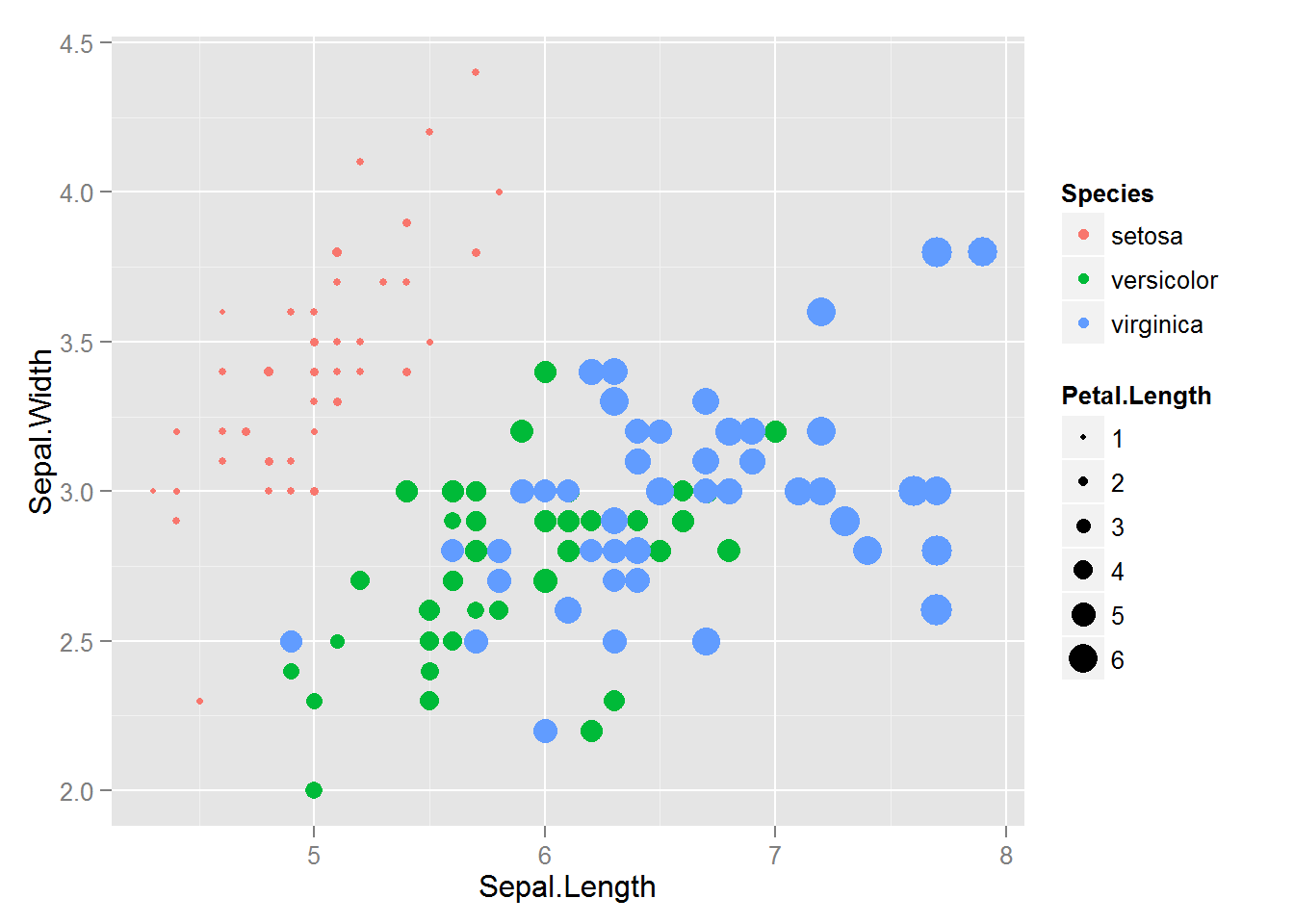#This looks a little object-oriented like python
pyggplotly(gg) You can embed code like this (which you get from the plotly ‘share’ dialogue): <div> <a href="https://plot.ly/~jflournoy/16/" target="_blank" title="Sepal.Width vs Sepal.Length" style="display: block; text-align: center;"><img src="https://plot.ly/~jflournoy/16.png" alt="Sepal.Width vs Sepal.Length" style="max-width: 100%;width: 797px;" width="797" onerror="this.onerror=null;this.src='https://plot.ly/404.png';" /></a> <script data-plotly="jflournoy:16" src="https://plot.ly/embed.js" async></script> </div> You can also directly embed a plotly plot using a code chunk if you set plotly=TRUE for the chunk, and include session="knitr" in the call. #Set plotly=TRUE pyggplotly(gg, session="knitr")

There’s a wide world of plotly fun just waiting out there.

# R Graph Catalog

This is Shahar’s distance contribution to R Club. (Thanks, Shahar!)

This catalog allows you to choose which graph you like best and gives you the code for that graph. You can also filter by things like Good vs. Bad graphs, type of graph, and different features that you might like to include (like subscripts and multiple plots).

http://shinyapps.stat.ubc.ca/r-graph-catalog/

# LOOCV

LOOCV (leave one out cross validation)

Download this excellent book: http://www-bcf.usc.edu/~gareth/ISL/ and search “LOOCV” or just scroll to section 5.1.2. on p. 178.

Here’s the dataset we’re using: http://www-bcf.usc.edu/~gareth/ISL/Auto.csv

# LOOCV (leave one out cross validation) install.packages("boot") require(boot) ?cv.glm

 # cv.glm(data, glmfit, cost, K) # this runs k-fold cross validation. When k = the number of observations in your dataset, then that's LOOCV # to run LOOCV, set k=n or just don't specify (its default is k=n) # cost specifies how to evaluate how good the model is; the default is the average squared error function. # it requires a glm object as input, so you need to run your model first and then validate it. 

Auto <- read.csv("http://www-bcf.usc.edu/~gareth/ISL/Auto.csv") str(Auto) # uh-oh. it interpreted horsepower as a factor, but it shoudl be numeric. Auto$horsepower <- as.numeric(Auto$horsepower) # run a simple regression, predicting mpg from horsepower model1 <- glm(mpg ~ horsepower, data=Auto) summary(model1) # okay, now let's cross-validate that model. # note: this takes a long time! it's running nearly 400 models. if you want it to be faster, you can set k to something smaller than n loocv1 <- cv.glm(data=Auto, glmfit=model1) # the only thing you really need in this loocv is an object called delta. it's got two items in it. The first component is the raw cross-validation estimate of prediction error. The second component is the adjusted cross-validation estimate. The adjustment is designed to compensate for the bias introduced by not using leave-one-out cross-validation. Since we ran LOOCV, the two values should be super similar. loocv1$delta # okay great. we have an estimate of model fit for the simple linear regession. let's play around with adding polynomial terms and see if we can improve the fit. # there's a handy way to add polynomial terms using poly() model2 <- glm(mpg ~ poly(horsepower, 2), data=Auto) model3 <- glm(mpg ~ poly(horsepower, 3), data=Auto) model4 <- glm(mpg ~ poly(horsepower, 4), data=Auto) model5 <- glm(mpg ~ poly(horsepower, 5), data=Auto) model6 <- glm(mpg ~ poly(horsepower, 6), data=Auto) # i'm going to switch from LOOCV to a 20-fold cv, to save a little processing time squared_errs <- rep(0,6) loocv <- cv.glm(data=Auto, glmfit=model1, K=20) squared_errs <- loocv$delta loocv <- cv.glm(data=Auto, glmfit=model2, K=20) squared_errs <- loocv$delta loocv <- cv.glm(data=Auto, glmfit=model3, K=20) squared_errs <- loocv$delta loocv <- cv.glm(data=Auto, glmfit=model4, K=20) squared_errs <- loocv$delta loocv <- cv.glm(data=Auto, glmfit=model5, K=20) squared_errs <- loocv$delta loocv <- cv.glm(data=Auto, glmfit=model6, K=20) squared_errs <- loocv$delta # I'm sure there's a way better way to do that with a for loop or something, but i couldn't get it to work. # this is a vector of the squared error for each model squared_errs # let's plot it to get a sense for how fit changes as a function of polynomial order plot(squared_errs) # looks like there's a big drop in error when we go from 2nd order to 3rd order (i.e. allowing a cubic function) # that makes sense, given the data: plot(Auto$mpg~Auto$horsepower) # looks cubic # pretty plotting time :) library(ggplot2) library(RColorBrewer) ggplot(data=Auto, aes(x=horsepower, y=mpg)) + geom_point() # to plot the lines from each function, use predict() to get the predicted values from each regression equation Auto$model1 <- predict(model1) Auto$model2 <- predict(model2) Auto$model3 <- predict(model3) Auto$model4 <- predict(model4) Auto$model5 <- predict(model5) Auto$model6 <- predict(model6) # pick a palette display.brewer.all() colors <- brewer.pal(9,"Spectral") colors <- c(colors[1:3], colors[7:9]) #take just the 6 colors from the two ends (they're brighter) # how to check out a color palette n <- 6 # the number of colors to plot pie(rep(1,n), col=colors) # plot all of the 6 models on the data. notice that the model doesn't improve much after cubic. ggplot(data=Auto, aes(x=horsepower, y=mpg)) + geom_point() + geom_line(aes(x=horsepower, y=model1, colour="linear"), size = 2, alpha = .5 ) + geom_line(aes(x=horsepower, y=model2, colour="quadratic"), size = 2, alpha = .5 ) + geom_line(aes(x=horsepower, y=model3, colour="cubic"), size = 2, alpha = .5 ) + geom_line(aes(x=horsepower, y=model4, colour="quartic"), size = 2, alpha = .5 ) + geom_line(aes(x=horsepower, y=model5, colour="fiveish"), size = 2, alpha = .5 ) + geom_line(aes(x=horsepower, y=model6, colour="sixtacular"), size = 2, alpha = .5 ) + guides(color=guide_legend(title="model"))  # APA style charts in ggplot Check out this great page for examples of complex charts in APA style, with all the bells and whistles (adding weird types of legends, showing * for significance, etc.): http://egret.psychol.cam.ac.uk/statistics/R/graphs2.html It looks like some of the options may be little outdated, but it’s certainly a valuable resource regardless. # Simple slopes in ggplot2! For a recent assignment in Sanjay’s SEM class, we had to plot interactions between two continuous variables – the model was predicting students’ grades (GRADE) from how often they attend class (ATTEND) and how many of the assigned books they read (BOOKS), and their interaction. I did all the plotting in ggplot2. It was my first time trying to add lines for different categories to the same plot, and I really wanted labels for each line to show up in the plot legend, which was trickier than I would have thought. I got it to work, though! Here’s a link to an HTML version of my homework document, which includes Sanjay’s instructions, etc. For this assignment, you should download the dataset grade_read_attend.sav (linked above). The dataset is in SPSS format. (Credit for the dataset goes to Jeremy Miles.) This (made-up) dataset contains data from 40 students in a literature class. The dataset contains 3 variables: • GRADE is the student's final grade (out of 100) • BOOKS is the number of assigned books that the student actually read (out of 4) • ATTEND is the number of class meetings the student attended (out of 20) In your favorite statistical package (SPSS, SAS, R, whatever) do the following: Run a simple regression in which you regress GRADE on ATTEND. (In regressionspeak, you say “regress Y on X,” where Y is the dependent/response variable and X is the independent/input variable. Thus, I am telling you to treat GRADE as the dependent variable and ATTEND as the independent variable.) Using the output of your analysis, do the following: • (1a) Write out the algebraic equation representing this analysis, using the unstandardized coefficient estimates from your output (that is, write out the best-fitting linear model predicting GRADE from ATTEND). • (1b) Create a graph in which the Y-axis is GRADE and the X-axis is ATTEND. Draw a line representing the slope of ATTEND for a realistic range of values. (You can do this by hand, or using whatever software you'd like.) library(foreign) data <- read.spss("/Users/TARDIS/Downloads/grade_read_attend.sav", to.data.frame = TRUE) # Run a simple regression in which you regress GRADE on ATTEND model1 <- lm(GRADE ~ ATTEND, data = data) summary(model1)  ## ## Call: ## lm(formula = GRADE ~ ATTEND, data = data) ## ## Residuals: ## Min 1Q Median 3Q Max ## -27.78 -10.90 2.02 12.43 31.76 ## ## Coefficients: ## Estimate Std. Error t value Pr(>|t|) ## (Intercept) 36.998 8.169 4.53 5.7e-05 *** ## ATTEND 1.883 0.555 3.39 0.0016 ** ## --- ## Signif. codes: 0 '***' 0.001 '**' 0.01 '*' 0.05 '.' 0.1 ' ' 1 ## ## Residual standard error: 14.8 on 38 degrees of freedom ## Multiple R-squared: 0.233, Adjusted R-squared: 0.212 ## F-statistic: 11.5 on 1 and 38 DF, p-value: 0.00163   # Write out the algebraic equation representing this analysis print(paste("GRADE = ", round(model1$coeff, digits = 2), " + ", round(model1$coeff, digits = 2), "*ATTEND + e", sep = ""))  ##  "GRADE = 37 + 1.88*ATTEND + e"   # Create a graph in which the Y-axis is GRADE and the X-axis is ATTEND. Draw # a line representing the slope of ATTEND for a realistic range of values. library(ggplot2) ggplot(data, aes(x = ATTEND, y = GRADE)) + geom_point(shape = 1) + geom_smooth(method = lm, se = FALSE)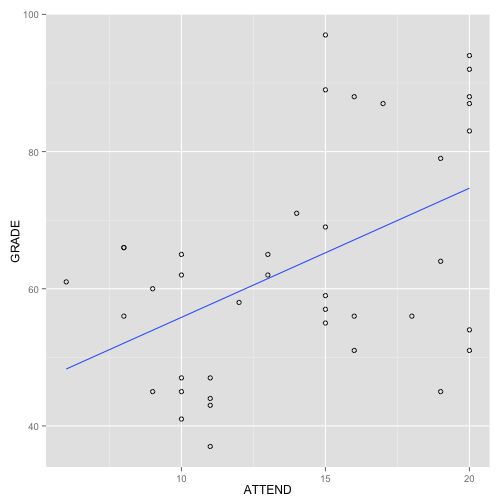Run a multiple regression in which you regress GRADE on BOOKS and ATTEND. Using the output of your analysis: • (2a) Write the algebraic equation for this analysis as above. • (2b) Create a graph with the same axes as 1b above. Graph the regression lines of ATTEND for students who have read 0, 2, and 4 books (so you should draw 3 lines).  # Run a multiple regression in which you regress GRADE on BOOKS and ATTEND model2 <- lm(GRADE ~ ATTEND + BOOKS, data = data) summary(model2)  ## ## Call: ## lm(formula = GRADE ~ ATTEND + BOOKS, data = data) ## ## Residuals: ## Min 1Q Median 3Q Max ## -20.80 -13.37 0.06 9.17 32.29 ## ## Coefficients: ## Estimate Std. Error t value Pr(>|t|) ## (Intercept) 37.379 7.745 4.83 2.4e-05 *** ## ATTEND 1.283 0.587 2.19 0.035 * ## BOOKS 4.037 1.753 2.30 0.027 * ## --- ## Signif. codes: 0 '***' 0.001 '**' 0.01 '*' 0.05 '.' 0.1 ' ' 1 ## ## Residual standard error: 14.1 on 37 degrees of freedom ## Multiple R-squared: 0.329, Adjusted R-squared: 0.292 ## F-statistic: 9.06 on 2 and 37 DF, p-value: 0.000628   # Write out the algebraic equation representing this analysis print(paste("GRADE = ", round(model2$coeff, digits = 2), " + ", round(model2$coeff, digits = 2), "*ATTEND", " + ", round(model2$coeff, digits = 2), "*BOOKS + e",
sep = ""))

##  "GRADE = 37.38 + 1.28*ATTEND + 4.04*BOOKS + e"


# Create a graph with the same axes as 1b above. Graph the regression lines
# of ATTEND for students who have read 0, 2, and 4 books (so you should draw
# 3 lines).
ggplot(data, aes(x = ATTEND, y = GRADE)) + geom_point(shape = 1) + geom_abline(aes(color = "0 books"),
intercept = model2$coeff, slope = model2$coeff, show_guide = TRUE) +
geom_abline(aes(color = "2 books"), intercept = model2$coeff + 2 * model2$coeff,
slope = model2$coeff, show_guide = TRUE) + geom_abline(aes(color = "4 books"), intercept = model2$coeff + 4 * model2$coeff, slope = model2$coeff,
show_guide = TRUE) + guides(color = guide_legend(title = "Number of books read"))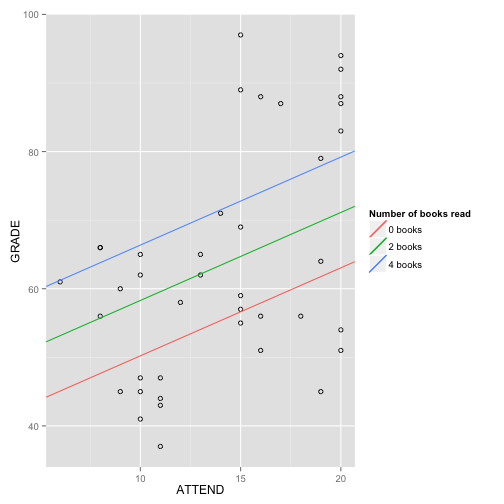Run a multiple regression in which you regress GRADE on BOOKS, ATTEND, and the interaction of BOOKS with ATTEND. Do not center any of the variables. Using the output of your analysis:

• (3a) Write the algebraic equation representing the results of this analysis three times. The first time, write it in standard form. The second time, rearrange it so that you can easily see the conditional slope of BOOKS. The third time, rearrange again so you can easily see the conditional slope of ATTEND.

• (3b) Draw a graph with GRADE on the Y-axis and ATTEND on the X-axis. Draw 3 lines depicting the regressions of ATTEND for students who have read 0, 2, and 4 books.

• (3c) Draw a graph with GRADE on the Y-axis and BOOKS on the X-axis. Draw 3 lines depicting the regressions of BOOKS for students who have attended 10, 15, and 20 times.


# Run a multiple regression in which you regress GRADE on BOOKS, ATTEND, and
# the interaction of BOOKS with ATTEND
model3 <- lm(GRADE ~ ATTEND * BOOKS, data = data)
summary(model3)

##
## Call:
## lm(formula = GRADE ~ ATTEND * BOOKS, data = data)
##
## Residuals:
##    Min     1Q Median     3Q    Max
## -24.84 -11.85   1.47   8.16  34.20
##
## Coefficients:
##              Estimate Std. Error t value Pr(>|t|)
## (Intercept)    55.221     11.262    4.90    2e-05 ***
## ATTEND         -0.137      0.878   -0.16    0.877
## BOOKS          -6.208      5.151   -1.21    0.236
## ATTEND:BOOKS    0.735      0.349    2.10    0.042 *
## ---
## Signif. codes:  0 '***' 0.001 '**' 0.01 '*' 0.05 '.' 0.1 ' ' 1
##
## Residual standard error: 13.4 on 36 degrees of freedom
## Multiple R-squared:  0.402,  Adjusted R-squared:  0.352
## F-statistic: 8.07 on 3 and 36 DF,  p-value: 0.000306

b0 <- round(model3$coeff, digits = 2) attend <- round(model3$coeff, digits = 2)
books <- round(model3$coeff, digits = 2) interaction <- round(model3$coeff, digits = 2)

# Write the algebraic equation representing the results of this analysis
# three times. The first time, write it in standard form.
print(paste("GRADE = ", b0, " + ", attend, "*ATTEND", " + ", books, "*BOOKS + ",
interaction, "*ATTEND*BOOKS + e", sep = ""))

##  "GRADE = 55.22 + -0.14*ATTEND + -6.21*BOOKS + 0.73*ATTEND*BOOKS + e"

# The second time, rearrange it so that you can easily see the conditional
# slope of BOOKS.
print(paste("GRADE = ", b0, " + ", attend, "*ATTEND", " + (", books, " + ",
interaction, "*ATTEND)*BOOKS + e", sep = ""))

##  "GRADE = 55.22 + -0.14*ATTEND + (-6.21 + 0.73*ATTEND)*BOOKS + e"

# The third time, rearrange again so you can easily see the conditional
# slope of ATTEND.
print(paste("GRADE = ", b0, " + (", attend, " + ", interaction, "*BOOKS)*ATTEND + ",
books, "*BOOKS + e", sep = ""))

##  "GRADE = 55.22 + (-0.14 + 0.73*BOOKS)*ATTEND + -6.21*BOOKS + e"



# Draw a graph with GRADE on the Y-axis and ATTEND on the X-axis. Draw 3
# lines depicting the regressions of ATTEND for students who have read 0, 2,
# and 4 books.
ggplot(data, aes(x = ATTEND, y = GRADE)) + geom_point(shape = 1) + geom_abline(aes(color = "0 books"),
intercept = b0, slope = attend, show_guide = TRUE) + geom_abline(aes(color = "2 books"),
intercept = b0 + 2 * books, slope = attend + 2 * interaction, show_guide = TRUE) +
geom_abline(aes(color = "4 books"), intercept = b0 + 4 * books, slope = attend +
4 * interaction, show_guide = TRUE) + guides(color = guide_legend(title = "Number of books read"))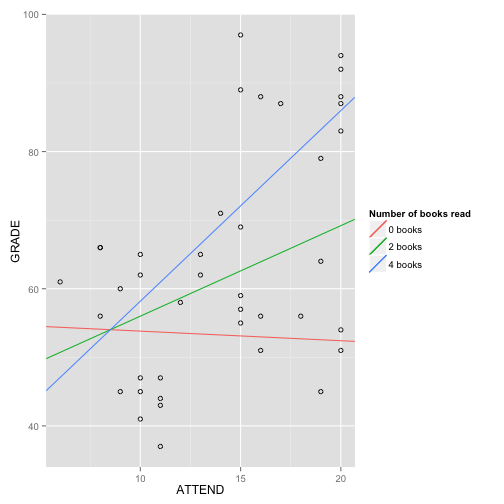# Draw a graph with GRADE on the Y-axis and BOOKS on the X-axis. Draw 3
# lines depicting the regressions of BOOKS for students who have attended
# 10, 15, and 20 times.
ggplot(data, aes(x = BOOKS, y = GRADE)) + geom_point(shape = 1) + geom_abline(aes(color = "Attended 10 times"),
intercept = b0 + 10 * attend, slope = books + 10 * interaction, show_guide = TRUE) +
geom_abline(aes(color = "Attended 15 times"), intercept = b0 + 15 * attend,
slope = books + 15 * interaction, show_guide = TRUE) + geom_abline(aes(color = "Attended 20 times"),
intercept = b0 + 20 * attend, slope = books + 20 * interaction, show_guide = TRUE) +
guides(color = guide_legend(title = "Number of classes attended"))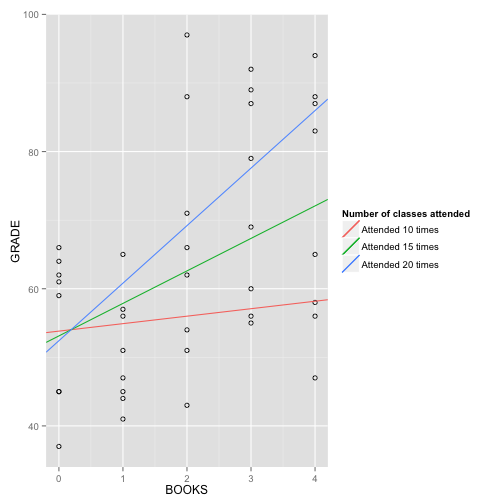Repeat the analysis you ran for #3, only this time you should first center BOOKS and ATTEND around their means, and then regress GRADE on BOOKS(centered), ATTEND(centered), and their interaction.

• (4a) Write the algebraic equation representing the results of this analysis three times. The first time, write it in standard form. The second time, rearrange it so that you can easily see the conditional slope of BOOKS. The third time, rearrange again so you can easily see the conditional slope of ATTEND.

• (4b) Draw a graph with GRADE on the Y-axis and ATTEND on the X-axis. Draw 3 lines depicting the regressions of ATTEND for students who have read 0, 2, and 4 books.

• (4c) Draw a graph with GRADE on the Y-axis and BOOKS on the X-axis. Draw 3 lines depicting the regressions of BOOKS for students who have attended 10, 15, and 20 times.

data$ATTENDc <- data$ATTEND - mean(data$ATTEND) data$BOOKSc <- data$BOOKS - mean(data$BOOKS)

# Run a multiple regression in which you regress GRADE on BOOKS, ATTEND, and
# the interaction of BOOKS with ATTEND
model4 <- lm(GRADE ~ ATTENDc * BOOKSc, data = data)
summary(model4)

##
## Call:
## lm(formula = GRADE ~ ATTENDc * BOOKSc, data = data)
##
## Residuals:
##    Min     1Q Median     3Q    Max
## -24.84 -11.85   1.47   8.16  34.20
##
## Coefficients:
##                Estimate Std. Error t value Pr(>|t|)
## (Intercept)      61.602      2.319   26.57   <2e-16 ***
## ATTENDc           1.333      0.562    2.37    0.023 *
## BOOKSc            4.155      1.678    2.48    0.018 *
## ATTENDc:BOOKSc    0.735      0.349    2.10    0.042 *
## ---
## Signif. codes:  0 '***' 0.001 '**' 0.01 '*' 0.05 '.' 0.1 ' ' 1
##
## Residual standard error: 13.4 on 36 degrees of freedom
## Multiple R-squared:  0.402,  Adjusted R-squared:  0.352
## F-statistic: 8.07 on 3 and 36 DF,  p-value: 0.000306

b0 <- round(model4$coeff, digits = 2) attend <- round(model4$coeff, digits = 2)
books <- round(model4$coeff, digits = 2) interaction <- round(model4$coeff, digits = 2)

# Write the algebraic equation representing the results of this analysis
# three times. The first time, write it in standard form.
print(paste("GRADE = ", b0, " + ", attend, "*ATTENDc", " + ", books, "*BOOKSc + ",
interaction, "*ATTENDc*BOOKSc + e", sep = ""))

##  "GRADE = 61.6 + 1.33*ATTENDc + 4.15*BOOKSc + 0.73*ATTENDc*BOOKSc + e"

# The second time, rearrange it so that you can easily see the conditional
# slope of BOOKSc.
print(paste("GRADE = ", b0, " + ", attend, "*ATTENDc", " + (", books, " + ",
interaction, "*ATTENDc)*BOOKSc + e", sep = ""))

##  "GRADE = 61.6 + 1.33*ATTENDc + (4.15 + 0.73*ATTENDc)*BOOKSc + e"

# The third time, rearrange again so you can easily see the conditional
# slope of ATTENDc.
print(paste("GRADE = ", b0, " + (", attend, " + ", interaction, "*BOOKSc)*ATTENDc + ",
books, "*BOOKSc + e", sep = ""))

##  "GRADE = 61.6 + (1.33 + 0.73*BOOKSc)*ATTENDc + 4.15*BOOKSc + e"



# Draw a graph with GRADE on the Y-axis and ATTENDc on the X-axis. Draw 3
# lines depicting the regressions of ATTENDc for students who have read 0,
# 2, and 4 booksc.
books0c <- 0 - mean(data$BOOKS) books2c <- 2 - mean(data$BOOKS)
books4c <- 4 - mean(data$BOOKS) ggplot(data, aes(x = ATTENDc, y = GRADE)) + geom_point(shape = 1) + geom_abline(aes(color = "0 books"), intercept = (b0 + books0c * books), slope = (attend + books0c * interaction), show_guide = TRUE) + geom_abline(aes(color = "2 books"), intercept = (b0 + books2c * books), slope = (attend + books2c * interaction), show_guide = TRUE) + geom_abline(aes(color = "4 books"), intercept = (b0 + books4c * books), slope = (attend + books4c * interaction), show_guide = TRUE) + guides(color = guide_legend(title = "Number of books read"))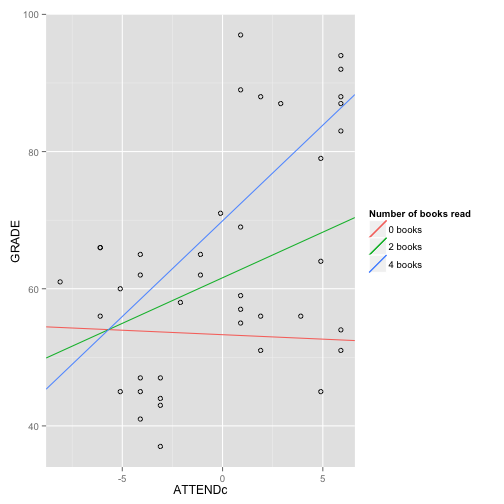# Draw a graph with GRADE on the Y-axis and BOOKS on the X-axis. Draw 3 # lines depicting the regressions of BOOKS for students who have attended # 10, 15, and 20 times. attend10c <- 10 - mean(data$ATTEND)
attend15c <- 15 - mean(data$ATTEND) attend20c <- 20 - mean(data$ATTEND)

ggplot(data, aes(x = BOOKSc, y = GRADE)) + geom_point(shape = 1) + geom_abline(aes(color = "Attended 10 times"),
intercept = (b0 + attend10c * attend), slope = (books + attend10c * interaction),
show_guide = TRUE) + geom_abline(aes(color = "Attended 15 times"), intercept = (b0 +
attend15c * attend), slope = (books + attend15c * interaction), show_guide = TRUE) +
geom_abline(aes(color = "Attended 20 times"), intercept = (b0 + attend20c *
attend), slope = (books + attend20c * interaction), show_guide = TRUE) +
guides(color = guide_legend(title = "Number of classes attended"))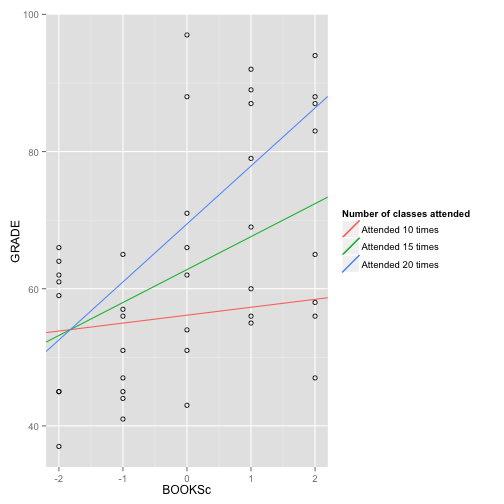Repeat #3, only this time you should first z-score all three variables (GRADE, BOOKS, and ATTEND), and then run the regression on the z-scored variables, including the product of z-BOOKS times z-ATTEND.

• (5a) Write the algebraic equation representing the results of this analysis three times. The first time, write it in standard form. The second time, rearrange it so that you can easily see the conditional slope of BOOKS. The third time, rearrange again so you can easily see the conditional slope of ATTEND.

• (5b) Draw a graph with GRADE on the Y-axis and ATTEND on the X-axis. Draw 3 lines depicting the regressions of ATTEND: one line for students who have read an average number of books, one line for students whose value on BOOKS is 1 standard deviation below the mean, and one line for students whose value on BOOKS is 1 standard deviation above the mean.

• (5c) Draw a graph with GRADE on the Y-axis and BOOKS on the X-axis. Draw 3 lines depicting the regressions of BOOKS: one line for students who have attended an average number of times, one line for students whose attendance is 1 standard deviation below the mean, and one line for students whose attendance is 1 standard deviation above the mean.

data$ATTENDz <- (data$ATTEND - mean(data$ATTEND))/sd(data$ATTEND)
data$BOOKSz <- (data$BOOKS - mean(data$BOOKS))/sd(data$BOOKS)

# Run a multiple regression in which you regress GRADE on BOOKSz, ATTENDz,
# and the interaction of BOOKSz with ATTENDz
model5 <- lm(GRADE ~ ATTENDz * BOOKSz, data = data)
summary(model5)

##
## Call:
## lm(formula = GRADE ~ ATTENDz * BOOKSz, data = data)
##
## Residuals:
##    Min     1Q Median     3Q    Max
## -24.84 -11.85   1.47   8.16  34.20
##
## Coefficients:
##                Estimate Std. Error t value Pr(>|t|)
## (Intercept)       61.60       2.32   26.57   <2e-16 ***
## ATTENDz            5.70       2.40    2.37    0.023 *
## BOOKSz             5.95       2.40    2.48    0.018 *
## ATTENDz:BOOKSz     4.50       2.14    2.10    0.042 *
## ---
## Signif. codes:  0 '***' 0.001 '**' 0.01 '*' 0.05 '.' 0.1 ' ' 1
##
## Residual standard error: 13.4 on 36 degrees of freedom
## Multiple R-squared:  0.402,  Adjusted R-squared:  0.352
## F-statistic: 8.07 on 3 and 36 DF,  p-value: 0.000306

b0 <- round(model5$coeff, digits = 2) attend <- round(model5$coeff, digits = 2)
books <- round(model5$coeff, digits = 2) interaction <- round(model5$coeff, digits = 2)

# Write the algebraic equation representing the results of this analysis
# three times. The first time, write it in standard form.
print(paste("GRADE = ", b0, " + ", attend, "*ATTENDz", " + ", books, "*BOOKSz + ",
interaction, "*ATTENDz*BOOKSz + e", sep = ""))

##  "GRADE = 61.6 + 5.7*ATTENDz + 5.95*BOOKSz + 4.5*ATTENDz*BOOKSz + e"

# The second time, rearrange it so that you can easily see the conditional
# slope of BOOKSz.
print(paste("GRADE = ", b0, " + ", attend, "*ATTENDz", " + (", books, " + ",
interaction, "*ATTENDz)*BOOKSz + e", sep = ""))

##  "GRADE = 61.6 + 5.7*ATTENDz + (5.95 + 4.5*ATTENDz)*BOOKSz + e"

# The third time, rearrange again so you can easily see the conditional
# slope of ATTENDz.
print(paste("GRADE = ", b0, " + (", attend, " + ", interaction, "*BOOKSz)*ATTENDz + ",
books, "*BOOKSz + e", sep = ""))

##  "GRADE = 61.6 + (5.7 + 4.5*BOOKSz)*ATTENDz + 5.95*BOOKSz + e"



# Draw a graph with GRADE on the Y-axis and ATTEND on the X-axis. Draw 3
# lines depicting the regressions of ATTEND: one line for students who have
# read an average number of books, one line for students whose value on
# BOOKS is 1 standard deviation below the mean, and one line for students
# whose value on BOOKS is 1 standard deviation above the mean.
ggplot(data, aes(x = ATTENDz, y = GRADE)) + geom_point(shape = 1) + geom_abline(aes(color = "low books (-1SD)"),
intercept = b0 + -1 * books, slope = attend + -1 * interaction, show_guide = TRUE) +
geom_abline(aes(color = "ave. books (mean)"), intercept = b0, slope = attend,
show_guide = TRUE) + geom_abline(aes(color = "high books (+1SD)"), intercept = b0 +
1 * books, slope = attend + 1 * interaction, show_guide = TRUE) + guides(color = guide_legend(title = "Number of books read"))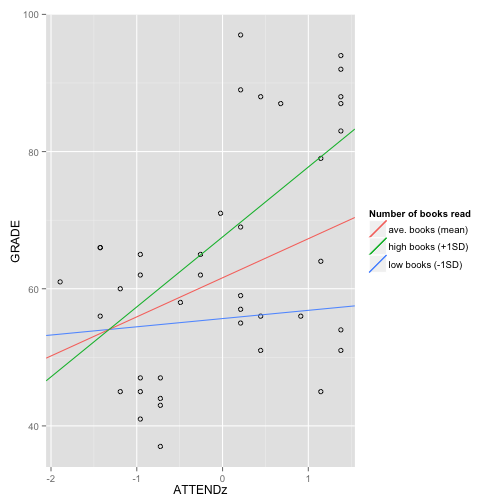# Draw a graph with GRADE on the Y-axis and BOOKS on the X-axis. Draw 3
# lines depicting the regressions of BOOKS: one line for students who have
# attended an average number of times, one line for students whose
# attendance is 1 standard deviation below the mean, and one line for
# students whose attendance is 1 standard deviation above the mean.
ggplot(data, aes(x = BOOKSz, y = GRADE)) + geom_point(shape = 1) + geom_abline(aes(colour = "low attendance (-1SD)"),
intercept = b0 + -1 * attend, slope = books + -1 * interaction, size = 1,
fullrange = T, show_guide = TRUE) + geom_abline(aes(colour = "ave. attendance (mean)"),
intercept = b0, slope = books, size = 1, fullrange = T, show_guide = TRUE) +
geom_abline(aes(colour = "high attendance (+1SD)"), intercept = b0 + 1 *
attend, slope = books + 1 * interaction, size = 1, fullrange = T, show_guide = TRUE) +
guides(colour = guide_legend(title = "Attendance"))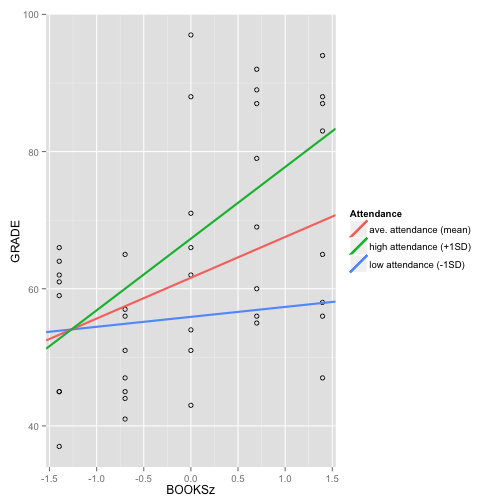Dataset source: http://www.jeremymiles.co.uk/regressionbook/data/

And John has another great way to do simple slopes in ggplot2!

I wanted to share this way of doing the simple slopes using the 'predict' function. This also demonstrates how to produce data on the fly -- good for reproducible examples!

#Replace this with your data. # For now, making up new stuff. summary(books<-round(runif(100,from=0,to=4),0)) #Get number of books from a uniform distribution from 0-4 summary(attend<-rnorm(100,mean=14,sd=4.3)) #Get the number of days attended from a normal distribution summary(grade<-55-.137*attend-6.2*books+.74*attend*books+ #The grade is related to books and attend... rnorm(100,0,20)) #...plus unmeasured things!

attend=attend,

#Update below is cool, but not necessary. It's just
# an easy way to make nested models. Maybe you've
# come across something like this or better?
summary(mod3<-update(mod1,.~.+books+attend:books))

#Uses a model to get predicted values for each row –
# you can use the original data or new data

require(plyr) #for the '.' function
require(ggplot2)
#I subset the data for geom_line or else we get a line for every value of books
geom_line(subset= .(books %in% c(0,2,4)), aes(colour = as.factor(books)), size = 1)+
# data. So honest!

# Plots!

Send in the coolest (or most useful, if you want to be practical about it) plot you can find. If you send it to me (rosem@uoregon.edu), I can add it to this post, or you can just include it in a comment.

So far, Paul is winning (see p. 81-83, and check out the R code on p. 123):
Correlating Maps (Yeah, that’s right – you can correlate maps.)

A much simpler plot, but still really lovely (check out the gorgeous semi-transparent overlapping!):
linear profiles with error envelopes (code)

And plotting networks!
visualizing networks

Bump plots, great for showing rank changes over time (includes some R Shiny!)
Bumps charts

Animated plots, both in 2D and 3D. Pretty bad ass.
http://alstatr.blogspot.com/2014/02/r-animating-2d-and-3d-plots.html

Plotting ggplot in plotly (great way to make website-friendly plots!)
http://ropensci.org/blog/2014/04/17/plotly/

Arc plots (in this case, showing network connections between Star Wars characters)
post
step-by-step description of how to make arc plots
R code

Some awesome plots via Matt:
I do really like the map visuals people have created, though, with packages like ggmap. Here is a map of Twitter activity in Europe done in R.